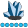plf515
Lapis Lazuli | Level 10

## How can I create a .png file with gridded output?

I am using SAS 9.4 1M6 on a Windows 10 machine.  I have a program that creates what I want in HTML:

``````ods listing gpath = "c:\writing\nonfiction\glm book";
ods graphics /imagename = "anscombe1sas" imagefmt = png;
ods layout gridded columns = 2 rows = 2;
ods region;
proc sgplot data = anscombe;
scatter x = x1 y = y1;
lineparm x = 0 y = 3.000090909 slope = 0.500090909/curvelabel = "OLS fit";
lineparm x = 0 y = 3.24 slope = 0.48/curvelabel = "Quantreg for median" lineattrs = (color = "red");
run;

ods region;
proc sgplot data = anscombe;
scatter x = x1 y = y2;
lineparm x = 0 y = 3.000909091 slope = 0.5/curvelabel = "OLS fit";
lineparm x = 0 y = 3.13 slope = 0.5/curvelabel = "Quantreg for median" lineattrs = (color = "red");
run;

ods region;
proc sgplot data = anscombe;
scatter x = x1 y = y3;
lineparm x = 0 y = 3.002454545 slope = 0.5/curvelabel = "OLS fit";
lineparm x = 0 y = 4.01 slope = 0.345/curvelabel = "Quantreg for median" lineattrs = (color = "red");
run;

ods region;
proc sgplot data = anscombe;
scatter x = x1 y = y4;
lineparm x = 0 y = 9.231363636 slope = -0.192272727/curvelabel = "OLS fit";
lineparm x = 0 y = 6.8067 slope = 0.0167/curvelabel = "Quantreg for median" lineattrs = (color = "red");
run;

ods layout end;``````

but PNG doesn't support gridded output, so there are multiple .png files. How can I create a single PNG file with 4 graphs?

1 ACCEPTED SOLUTION

Accepted SolutionsDanH_sas
SAS Super FREQ

## Re: How can I create a .png file with gridded output?

You'll need to use GTL instead. A LAYOUT LATTICE gives you the ability to put multiple plots in the same graph. The code below should get you close to what you want.

Hope it helps!

Dan

``````proc template;
define statgraph ScatPlots;
begingraph;
layout lattice / rows=2 columns=2;
layout overlay;
scatterplot x = x1 y = y1;
lineparm x = 0 y = 3.000090909 slope = 0.500090909/curvelabel = "OLS fit";
lineparm x = 0 y = 3.24 slope = 0.48/curvelabel = "Quantreg for median" lineat
trs = (color = red);
endlayout;
layout overlay;
scatterplot x = x1 y = y2;
lineparm x = 0 y = 3.000909091 slope = 0.5/curvelabel = "OLS fit";
lineparm x = 0 y = 3.13 slope = 0.5/curvelabel = "Quantreg for median" lineatt
rs = (color = red);
endlayout;
layout overlay;
scatterplot x = x1 y = y3;
lineparm x = 0 y = 3.002454545 slope = 0.5/curvelabel = "OLS fit";
lineparm x = 0 y = 4.01 slope = 0.345/curvelabel = "Quantreg for median" linea
ttrs = (color = red);
endlayout;
layout overlay;
scatterplot x = x1 y = y4;
lineparm x = 0 y = 9.231363636 slope = -0.192272727/curvelabel = "OLS fit";
lineparm x = 0 y = 6.8067 slope = 0.0167/curvelabel = "Quantreg for median" li
neattrs = (color = red);
endlayout;
endlayout;
endgraph;
end;
run;

proc sgrender data=anscombe template=ScatPlots;
run;``````DanH_sas
SAS Super FREQ

## Re: How can I create a .png file with gridded output?

You'll need to use GTL instead. A LAYOUT LATTICE gives you the ability to put multiple plots in the same graph. The code below should get you close to what you want.

Hope it helps!

Dan

``````proc template;
define statgraph ScatPlots;
begingraph;
layout lattice / rows=2 columns=2;
layout overlay;
scatterplot x = x1 y = y1;
lineparm x = 0 y = 3.000090909 slope = 0.500090909/curvelabel = "OLS fit";
lineparm x = 0 y = 3.24 slope = 0.48/curvelabel = "Quantreg for median" lineat
trs = (color = red);
endlayout;
layout overlay;
scatterplot x = x1 y = y2;
lineparm x = 0 y = 3.000909091 slope = 0.5/curvelabel = "OLS fit";
lineparm x = 0 y = 3.13 slope = 0.5/curvelabel = "Quantreg for median" lineatt
rs = (color = red);
endlayout;
layout overlay;
scatterplot x = x1 y = y3;
lineparm x = 0 y = 3.002454545 slope = 0.5/curvelabel = "OLS fit";
lineparm x = 0 y = 4.01 slope = 0.345/curvelabel = "Quantreg for median" linea
ttrs = (color = red);
endlayout;
layout overlay;
scatterplot x = x1 y = y4;
lineparm x = 0 y = 9.231363636 slope = -0.192272727/curvelabel = "OLS fit";
lineparm x = 0 y = 6.8067 slope = 0.0167/curvelabel = "Quantreg for median" li
neattrs = (color = red);
endlayout;
endlayout;
endgraph;
end;
run;

proc sgrender data=anscombe template=ScatPlots;
run;``````
Discussion stats